# Lecture 2 Implicit Differentiation Inverse Trigonometric Functions Higher

• Slides: 43Lecture 2: Implicit Differentiation, Inverse Trigonometric Functions, Higher Derivatives, Curve Sketching, and Min/Max ProblemsPart I: Exponentials, Implicit Differentiation, and Inverse Trigonometric Functions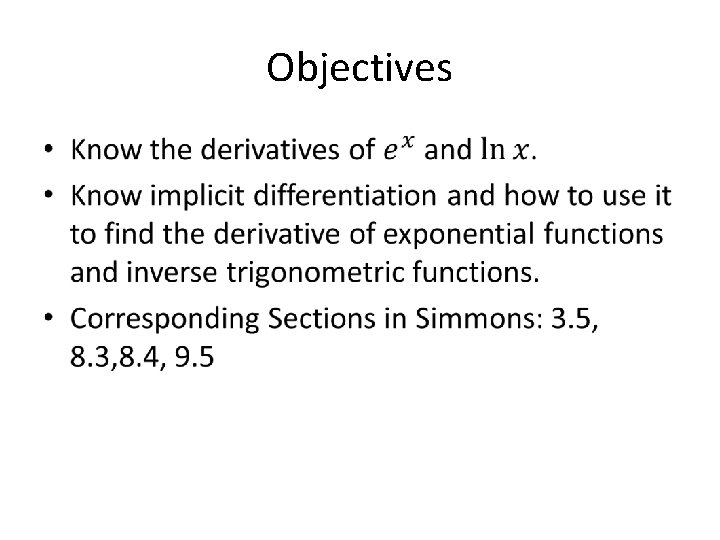Objectives •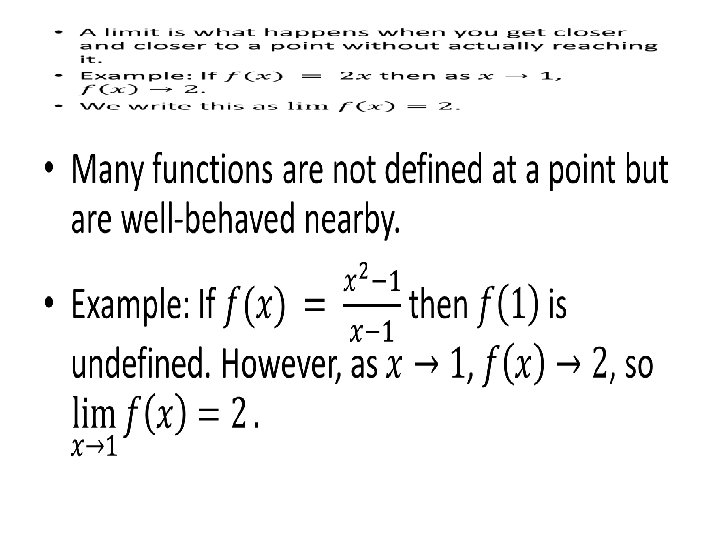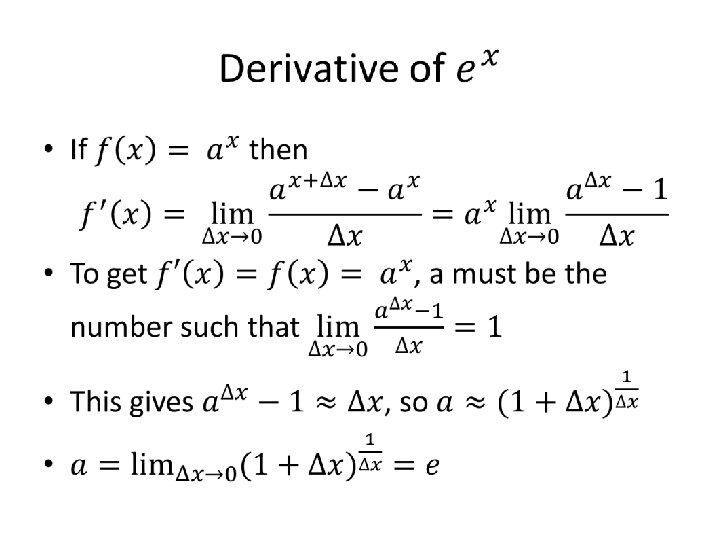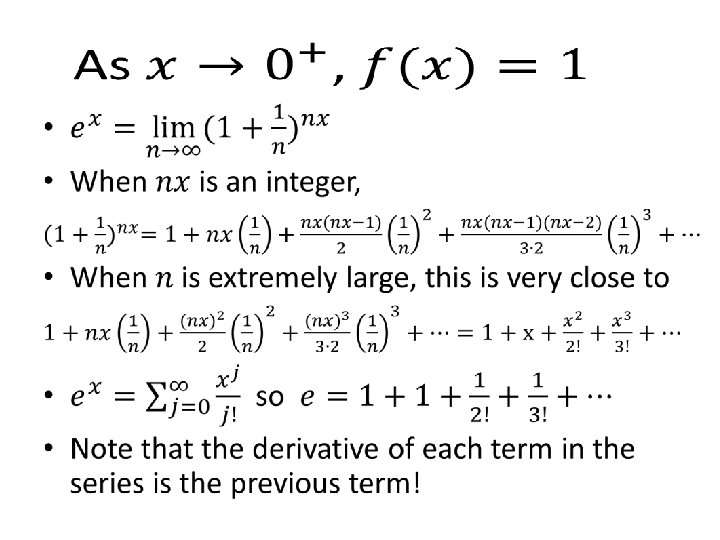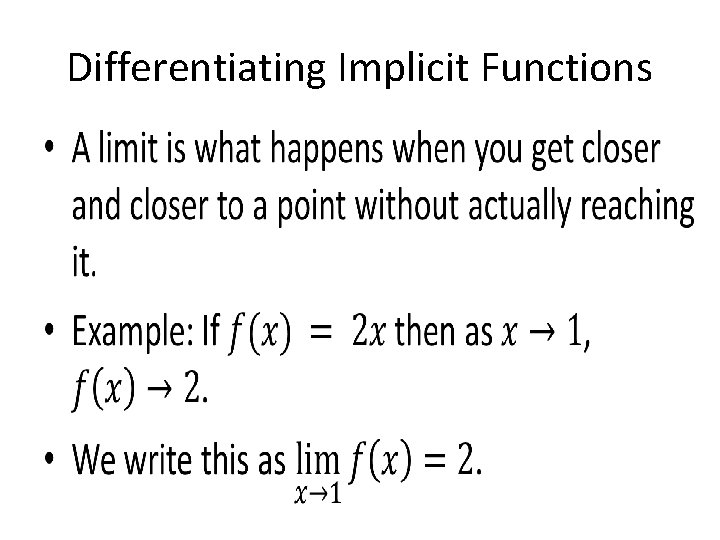Differentiating Implicit Functions •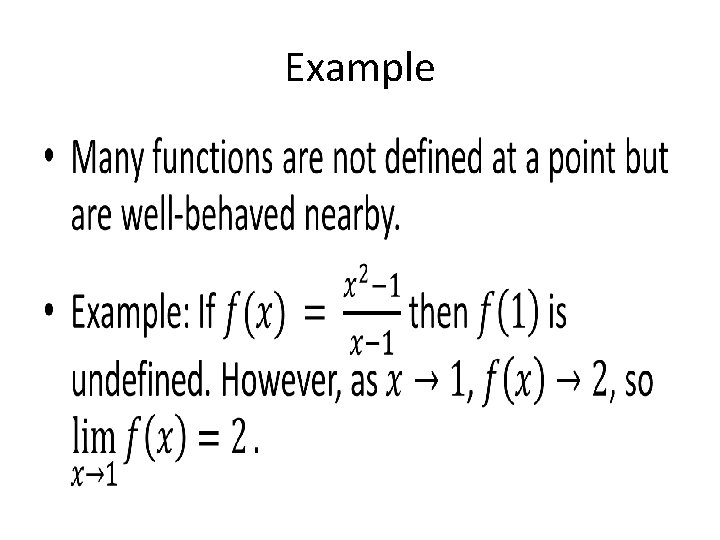Example •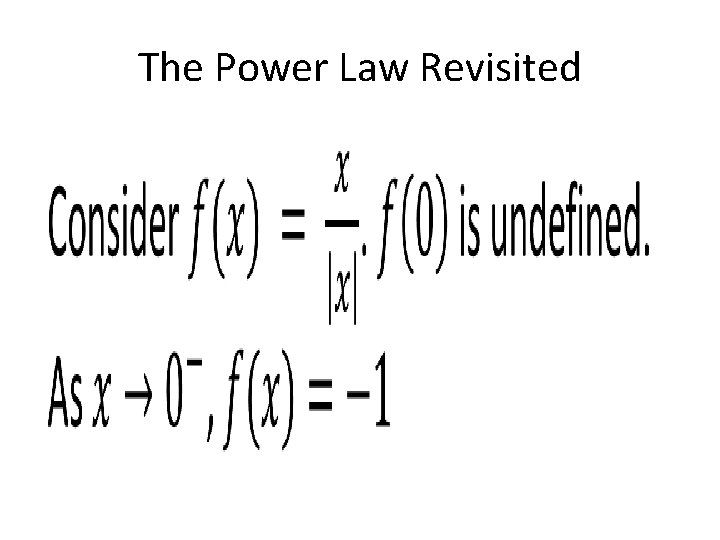The Power Law Revisited •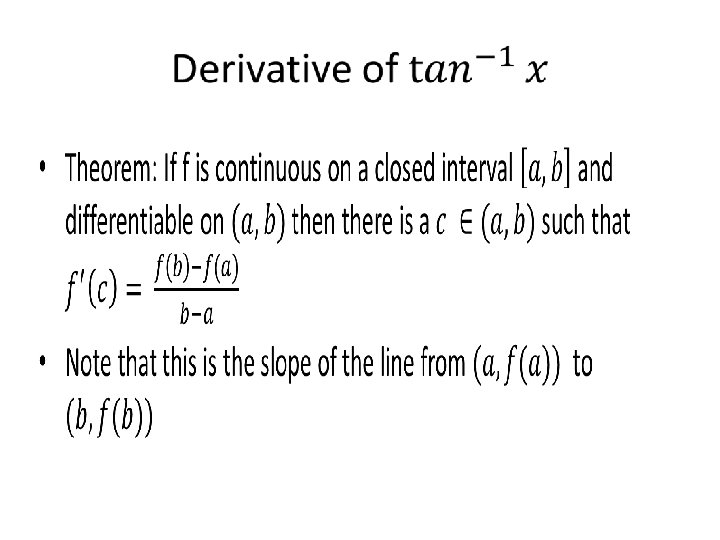Part II: Higher DerivativesThe Second Derivative •• The Second Derivative 4 3 2 1 0 -1 -2 -3 -4 -3 -2 -1 0 1 2 3 4 x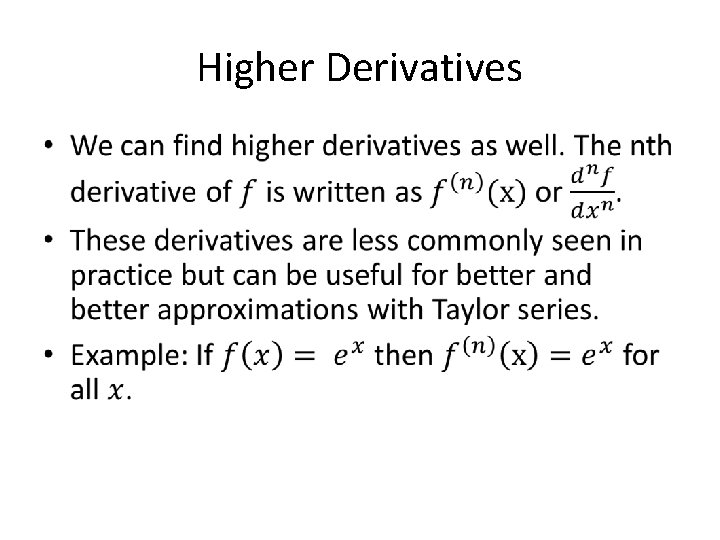Higher Derivatives •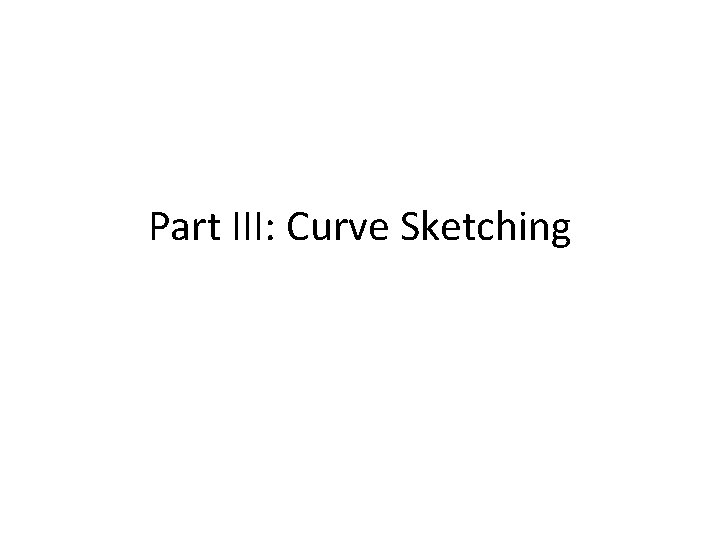Part III: Curve Sketching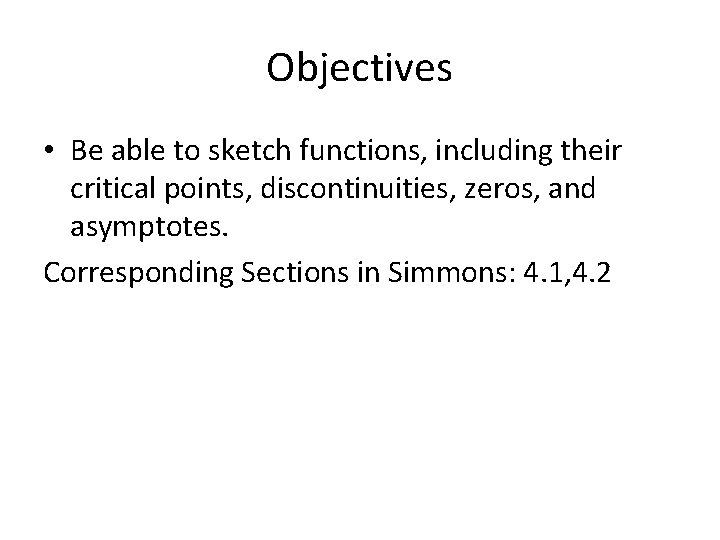Objectives • Be able to sketch functions, including their critical points, discontinuities, zeros, and asymptotes. Corresponding Sections in Simmons: 4. 1, 4. 2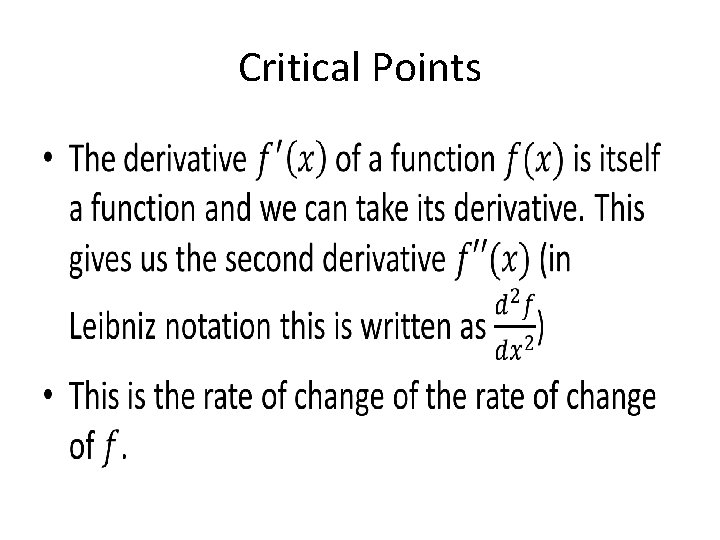Critical Points •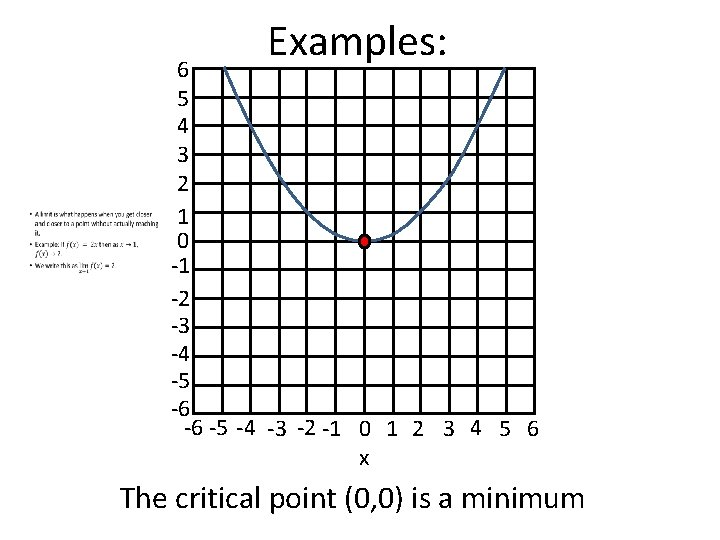Examples: 6 5 4 3 2 1 0 -1 -2 -3 -4 -5 -6 -6 -5 -4 -3 -2 -1 0 1 2 3 4 5 6 x The critical point (0, 0) is a minimumExamples: 6 5 4 3 2 1 0 -1 -2 -3 -4 -5 -6 -6 -5 -4 -3 -2 -1 0 1 2 3 4 5 6 x The critical point (0, 0) is not a minimum or maximumExamples: 6 5 4 3 2 1 0 -1 -2 -3 -4 -5 -6 -6 -5 -4 -3 -2 -1 0 1 2 3 4 5 6 x (0, 0) is a minimum but not a critical point.Information for Sketching Functions •• Examples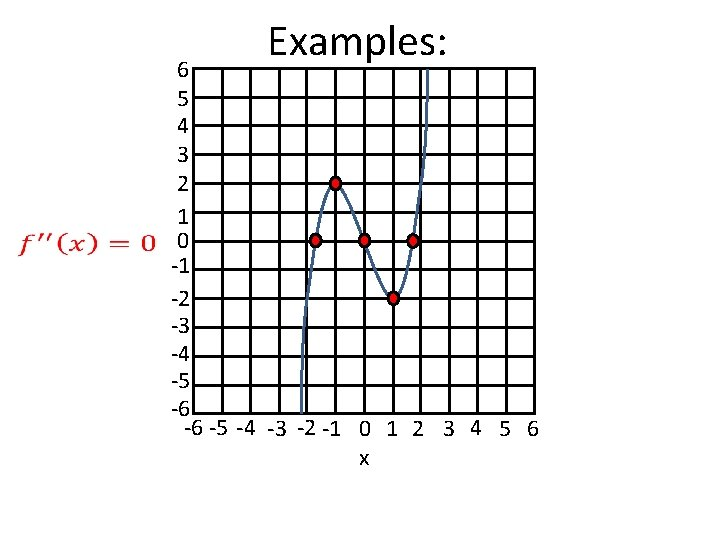Examples: 6 5 4 3 2 1 0 -1 -2 -3 -4 -5 -6 -6 -5 -4 -3 -2 -1 0 1 2 3 4 5 6 x• Examples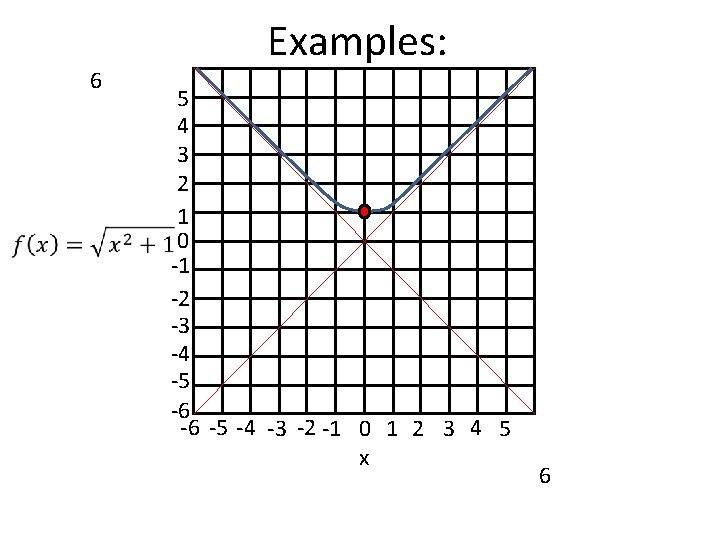6 Examples: 5 4 3 2 1 0 -1 -2 -3 -4 -5 -6 -6 -5 -4 -3 -2 -1 0 1 2 3 4 5 x 6• Examples6 Examples: 5 4 3 2 1 0 -1 -2 -3 -4 -5 -6 -6 -5 -4 -3 -2 -1 0 1 2 3 4 5 x 6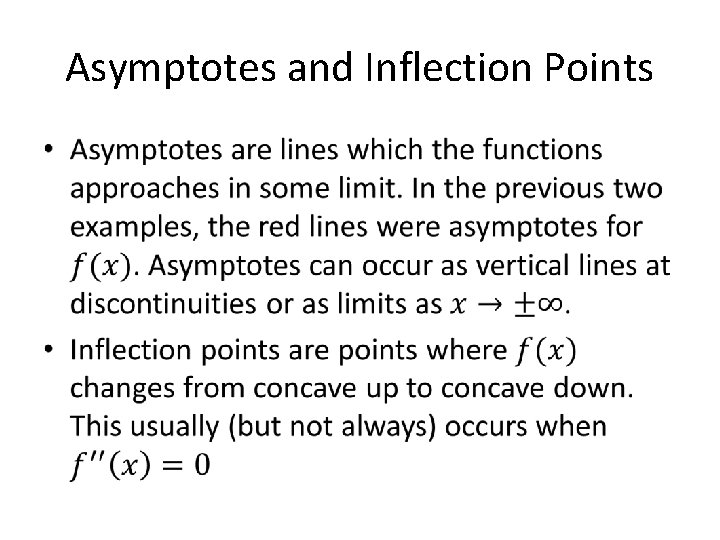Asymptotes and Inflection Points •Part IV: Min/Max Problems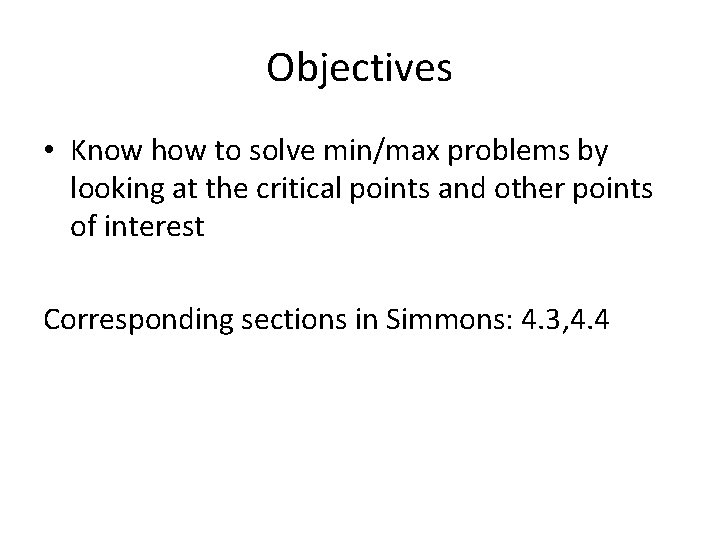Objectives • Know how to solve min/max problems by looking at the critical points and other points of interest Corresponding sections in Simmons: 4. 3, 4. 4Finding Absolute Minima/Maxima •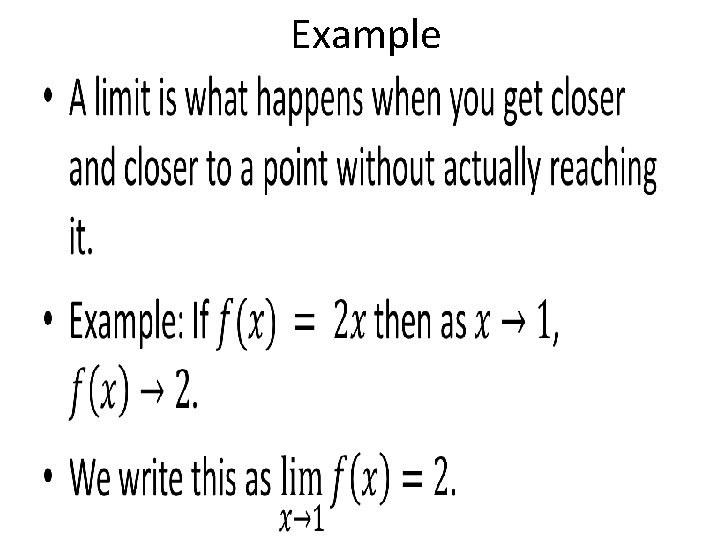• Example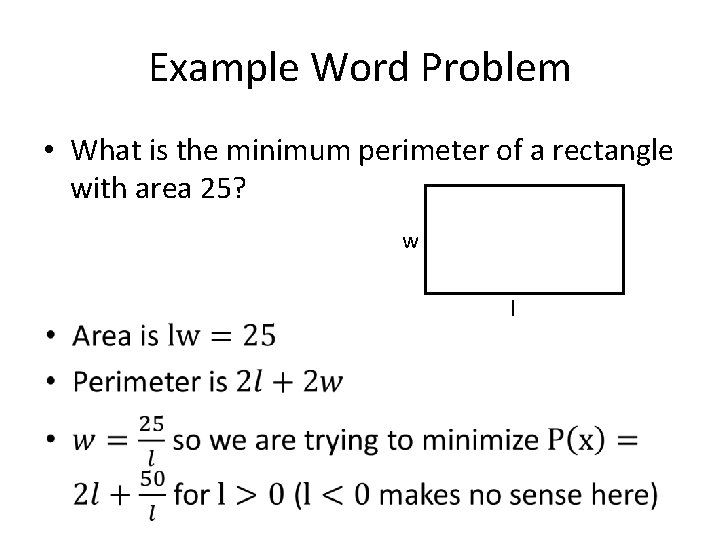Example Word Problem • What is the minimum perimeter of a rectangle with area 25? w lExample Word Problem Continued •Solving Word Problems • To solve word problems, we often: 1. Draw a picture for the problem 2. Find equations for the relevant variables 3. Use these equations to re-express what we’re trying to minimize/maximize as a function in one variable. 4. Minimize or maximize this function.The second derivative test •Reflection • What is the minimum length of a path from A to B that bounces off the mirror? A B a x b c-x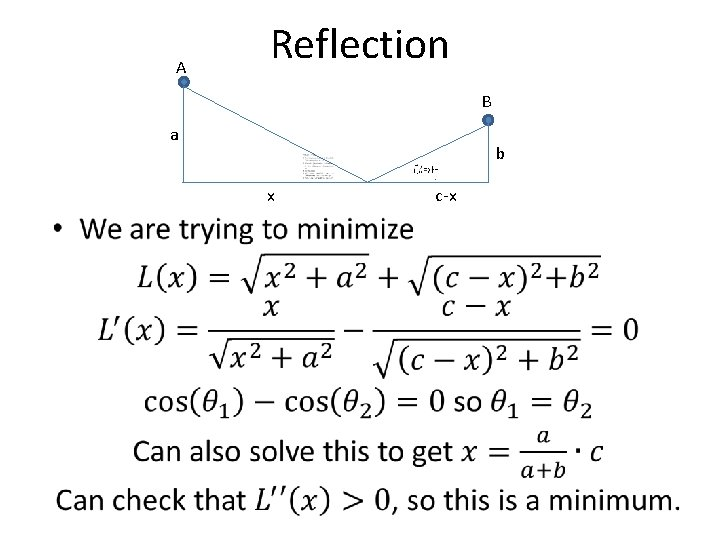A Reflection B a x b c-x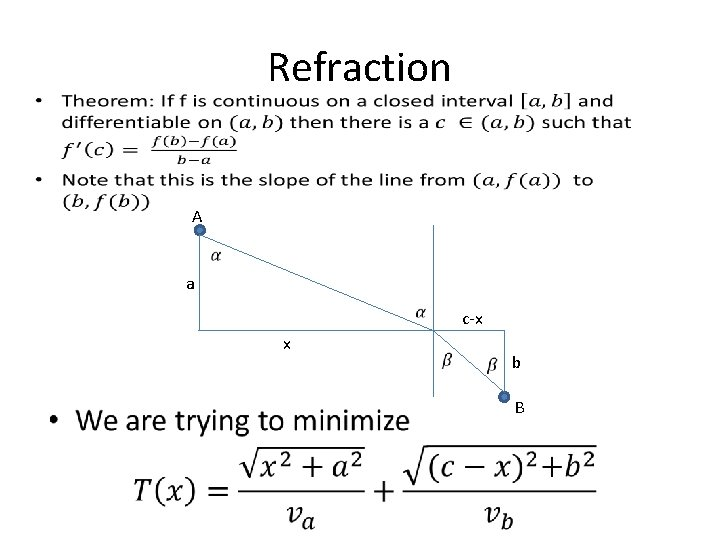Refraction • A a x c-x b B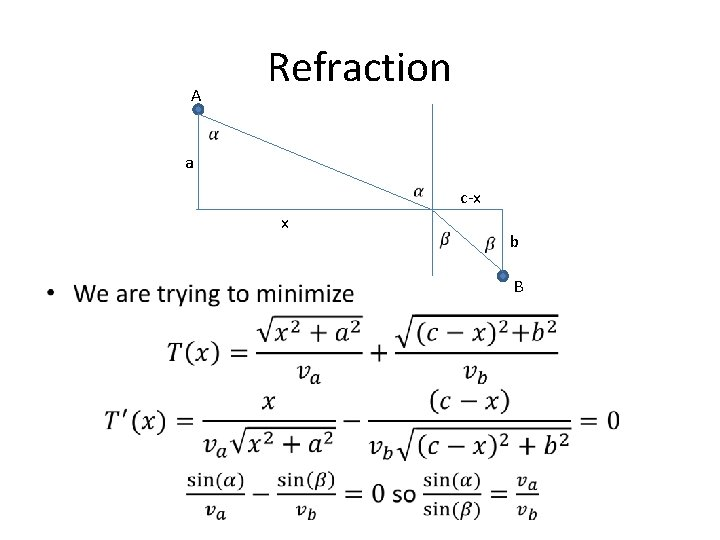Refraction A a x c-x b B